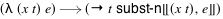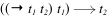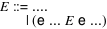## Redex @ SSLF

This document gives the Redex presentation at Summer School on Language Frameworks.

The slides from the introduction are available in pdf format.

The remainder of the document contains some exercises for you to work through to better learn Redex. Find a partner and do pair programming to try to solve them. (Pair programming means that the two of you sit together at the keyboard: one types and the other thinks and watches. Take turns in the two different roles.)

The Redex and Racket constructs used below in the running text are all linked to the online version of the documentation. You can also search in your local copy of the documentation for the same information without using the network by pressing f1.

To get started, visit the Racket website and install version 5.2.1 of Racket.

### 1Tutorial (optional)

If you wish to work through another tutorial-style presentation of Redex, but this time with you at the keyboard, please use the Amb Tutorial.

If you wish, however, skip the tutorial (but come back if you find the following assumes too much about Racket or Redex).

### 2Finger Exercises

All of the activities described here build on the same base language, the call-by-value λ-calculus. The grammar and reductions are shown in figure 1. (There are two auxiliary functions, Σ, which just adds two numbers, and subst-n, which performs non-capturing substitution.) The implementation for this language and its reduction relation, along with some example tests, are provided in the file cbv.rkt.Figure 1: Grammar and reductions for λv, the call-by-value lambda calculus

Exercise 1

The language in figure 1 is probably quite familiar. Compare it with the implementation in cbv.rkt and confirm that the implementation behaves as expected by coming up with a few example terms and viewing their reduction graphs using Redex’s traces function. To get started, open the file in DrRacket, hit the Run button, and enter (traces red (term ((λ (x num) (+ x x)) 3))) in the interactions window, and hit return. This should open a new where you can see the reduction sequence generated by that term.

View the documentation for traces, term, and reduction-relation by placing your cursor over the relevant identifier in DrRacket and typing F1. Generally speaking, the documentation contains information you will find relevant in solving the following exercises.

Read through the rest of the file and read up on the documentation of all of the Redex constructs used.

Exercise 2

The implementation of λv in cbv.rkt includes a number of test cases for the reduction relation red, written using test-->>. Formulate three new test cases and add them to cbv.rkt. Include one test case with at least two application expressions and two distinct variables.

Exercise 3

The redex-match form allows you to experiment with specific patterns in DrRacket’s interactions window. Use it to formulate a pattern that matches application expressions that have a λ-expression in their function positions.

For example, this expression matches (term ((λ (x num) x) 1)) but this one does not (term (f x)).

Use this pattern to write a function (using define-metafunction) that returns #t if such an expression appears anywhere in an expression. For example, (λ (x num) ((λ (x num) 2) 3)) would return #t, but (λ (f (-> num num)) (f 3)) would not.

Hint: You may find these functions helpful:
 (define-metafunction λv [(∧ #f any ...) #f] [(∧ #t any ...) (∧ any ...)] [(∧) #t]) (define-metafunction λv [(∨ #t any ...) #t] [(∨ #f any ...) (∨ any ...)] [(∨) #f])
You can type unicode symbols by typing the LaTeX command and then typing either control-\ or alt-\ (depending on the platform you are using). So, for ∨, type \vee and then the shortcut.

Exercise 4

Write a pattern (that can be used directly with redex-match) that matches exactly the expressions that the metafunction in the previous exercise would have matched.

Hint: use define-extended-language to extend λv with a new form of contexts.

Exercise 5

Use redex-check to check to see if the two formulations are, in fact, equivalent.

Remove a clause from the metafunction or a production from the context your defined and see if redex-check can discover a counter-example.

Exercise 6

Use define-judgment-form to reformulate the previous two exercises. Use redex-check again to make sure the new formulation is also equivalent to the others.

The documentation for define-judgment-form contains a number of examples to give you a feel for the syntax. If you wish, open a separate DrRacket window, put this at the top of the file
 #lang racket (require redex)
and try them out before solving this exercise.

Exercise 7Figure 2: λv-s, the call-by-value lambda calculus with mutable references, formed by extending λv as shown here.

To make things more interesting, we can add mutable references to our base calculus using the extensions shown in figure 2. The corresponding implementation is in cbv-s.rkt.

The new constructs are:
• box: creates a new, mutable cell that is initialized with the argument to box

• unbox: extracts the current value from a mutable cell

• set-box!: the first argument should be a mutable cell whose contents is updated with the value of the second argument; it returns the value that was in the cell before the mutation occurred

• begin: sequences two expressions, evaluating the first argument for its effect (discarding its result) and then evaluating the second argument

These new constructs match their counterparts in racket (except that begin is restricted to only two arguments and Racket’s set-box! returns (void)) and following the links takes you to their documentation in the Racket reference manual.

In Redex, an ellipsis with four periods, ...., indicates that we are adding productions to the non-terminals e, v, t, and to the context E. We also add a store Σ that maps locations l to values. The reduction relation now operates on programs p which pair a store with an expression. The store is used to hold the current values in the boxes and instead of treating (box v) as a value, box values are references to the store, written (* l).

Read over the content of cbv-s.rkt, looking up new constructs in the documentation. Try a few examples to be sure you have a feel for how expressions reduce.

Exercise 8

Formulate a function (in the object language) that accepts two (ref num) arguments and swaps their contents, returning the sum of the values in the ref cells.

Exercise 9

Define a variation of types (from cbv.rkt) that handles the new expressions in cbv-s.rkt.

Exercise 10

Extend λv and λv-s so that the functions accept multiple arguments. This will require using doubled ellipses in the evaluation contexts for application expressions.

### 3In-depth Exercises

Read over the beginning of the following sub-sections, pick one, and implement it. The subsections are independent of each other.

#### 3.1A Type System Via Reductions

Type systems are usually described via a relation specified with judgments as appears in cbv.rkt, but it is also possible to specify a type system using a reduction relation. This exercise explores that idea.

To get started, here is a rule that show how rewriting can capture type checking:This rule says that a function rewrites into an arrow type by pulling the domain type from the parameter and then putting the entire body of the λ expression as the co-domain, after substituting occurrences of the formal parameter. For example, the program (λ (x num) x) rewrites directly to its type by this rule, namely ( num num).

If the body of the function is more complex, however, more rewrites are needed. For example this function: (λ (f ( num num)) (f 1)) rewrites to ( ( num num) (( num num) 1)), a term that contains types and expressions co-mingled.

To cope with this term, additional rules are needed. For example, there should be a rule that rewrites numeric constants to the num type. To capture application expressions, we can use a rule that fires when the function position and argument position have already been rewritten to types (and the domain type of the function matches the type of the argument actually supplied):Exercise 1

These rules are incomplete. For example, they do not rewrite inside terms. And there is no rule for addition expressions yet.

Complete the rules by building a context where type rewriting can occur and by filling in enough rules to handle all of the operations in the language.

Hint: It is okay to extend the non-terminal e.

Write unit tests with test-->>, but also use redex-check to compare the rewriting-based type system to the traditional one already defined in cbv.rkt.

Exercise 2

Generalize your rewriting system to support type-checking expressions in λv-s. For this exercise, consider only expressions that do not contain values of the (* l) (and ignore the store).

Exercise 3

To use subject-reduction to prove that the λv-s type system works properly, you need a notion of type reduction that handles programs that have non-empty stores and use (* l) values.

Design one. Test it. Use it to formulate progress and preservation via the Racket functions progress-holds? and preservation-holds?, that each take a λv-s programs as an argument and return a boolean value. The predicate to test for progress should check that any well-typed term is either a value or reduces to some new term via the red-s relation. The predicate to test for preservation should check that when any well-typed term reduces to some new term via red-s, then both terms have the same type.

Warning: Be sure to explore the behavior of your type checker with cyclic references, e.g., (term (([l_1 (* l_1)]) (unbox (* l_1)))). Redex is smart enough to stop pursuing an infinite path that repeats, but beware that apply-reduction-relation* won’t terminate when the reduction graph is infinite (well, it will terminate when it runs out of memory...

#### 3.2Scheme’s unspecified order of evaluation

Scheme’s order of evaluation is unspecified, and we can use Redex’s reduction relations to model this behavior. (Note that Racket’s order of evaluation is, unlike Scheme’s, fully specified: left to right.)

Specifically, if the evalution context definition for application isthen, for example, this expression
 (((l:b 0)) ((λ (x y) (unbox (* l:b))) (set-box! (* l:b) 1) (set-box! (* l:b) 2)))
will reduce both to
 (((l:b 2)) ((λ (x y) (unbox (* l:b))) 0 (set-box! (* l:b) 1)))
and to:
 (((l:b 2)) ((λ (x y) (unbox (* l:b))) (set-box! (* l:b) 1) 0))
and these two expressions will ultimately produce different final results.

Unfortunately, this form of unspecified order of evaluation is too unspecified for Scheme. From the R6RS standard: “[a]lthough the order of evaluation is otherwise unspecified, the effect of any concurrent evaluation of the operator and operand expressions is constrained to be consistent with some sequential order of evaluation. The order of evaluation may be chosen differently for each procedure call.”

This means, for example, that this expression:
 (((l:b 0)) ((λ (x y) (unbox (* l:b))) (set-box! (* l:b) (+ (unbox (* l:b)) 1)) (set-box! (* l:b) (+ (unbox (* l:b)) 1))))
should reduce to 2 and to no other numbers because once it commits to evaluating either the first or second argument, it should finish evaluating that argument before moving to the other one.

Unfortunately, the context revision given above does not do that.

Exercise 1

Adjust the λv-s model with the evaluation context for application expressions given above. Use traces to try the example just above (that should reduce only to 2) and see what else it reduces to (and why).

Exercise 2

One way to faithfully model Scheme’s order of evaluation is to introduce a new form of expression that we call “marked” expressions, that can only appear in an application expression. We can exploit this form of expression by addding a non-deterministic reduction that reduces by inserting a mark into an application expression around some non-value (as long as there is not already a mark in the application) and only allow evaluation inside application expressions inside the mark.

Code up this idea and test it.

Exercise 3

It is also possible to model Scheme’s order of evaluation without introducing a new form of expression (i.e., without these explicitly “marked” expressions). Figure out how.